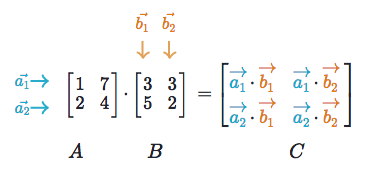# 1. What is dot prodcut?

The dot product is an algebraic operation that takes two same-sized vectors and returns a single number.

Algebraic definition
For two sequences of numbers, the dot product is the sum of the products of corresponding components of them. Think of two sequences $a$ and $b$ as below.

$a = \begin{bmatrix} a_1 & a_2 & ... & a_n \end{bmatrix} \\ b = \begin{bmatrix} b_1 & b_2 & ... & b_n \end{bmatrix} \\$

Then, the dot product of a and b becomes

$a \cdot b = \sum_{i=1}^{n} a_i b_i$

If $a$ and $b$ are row matrices, the dot product can be written as a matrix product. $a \cdot b = ab^\intercal$

For example, if $a = [a_1 \ a_2 \ a_3]$ and $b = [b_1 \ b_2 \ b_3]$, it becomes

$[a_1 \ a_2 \ a_3] \begin{bmatrix} b_1 \\ b_2 \\ b_3 \end{bmatrix} =a_1b_1 + a_2b_2 + a_3b_3$

Geometric definition
Geometrically, the dot product is the product of the Euclidean magnitudes of two vectors and the cosine of the angle between two.

$a \cdot b = \vert a \vert \vert b \vert \rm cos \theta$

Note that it is based on how much of one vector is in the direction of the other (projection). For example, in the below figure, the component of $A$ that is in the $B$ direction is $\vert A \vert \rm cos \theta$. Here, the magnitude of $A$ can be calculated by $\vert A \vert = \sqrt{x^2 + y^2}$ if $A = (x, y)$ and the initial point is the origin.Also note that if the two vectors are in the same direction, $\rm cos \theta = \rm cos 0^{\circ} = 1$ so it simply becomes the product of the magnitude of the two vectors, $a \cdot b = \vert a \vert \vert b \vert$. On the other hand, if the two vectors are perpendicular, the whole dot product becomes 0 because $\rm cos \theta = \rm cos 90^{\circ} = 0$.

Real world example
So what does the dot product really mean to us? How can we use it in the real life?
Imagine you are in a grocery store. You want to buy 1 apple, 2 oranges, and 3 bananas. The unit prices are \$1, \$2, \$0.5, respectively.You can define a number of items vector ($a$) and a unit price vector ($b$). $a = \begin{bmatrix}1 & 2 & 3 \end{bmatrix}\\ b = \begin{bmatrix}\1 & \2 & \0.5\end{bmatrix}\\$ The total cost will be the dot product of the two vectors: $ab^\intercal = \begin{bmatrix} 1 & 2 & 3 \end{bmatrix} \begin{bmatrix} \1 \\ \2 \\ \0.5 \end{bmatrix} =1 \times \1 + 2 \times \2 + 3 \times \0.5 = \6.5 \\$ Ta-da! Your total is$6.5! Now we understand the dot product is something useful in our life, right?

# 2. What is matrix multiplication?

Now that we know what the dot product is, let’s talk about matrix multiplication. How is it different from dot product?

Matrix multiplication is basically a matrix version of the dot product. Remember the result of dot product is a scalar. The result of matrix multiplication is a matrix, whose elements are the dot products of pairs of vectors in each matrix.Note that the number of columns in $A$ and the number of rows in $B$ should match; $A: (m \times n)$, $B: (n \times k)$.

Grocery example
Let’s go back to the previous grocery store example. Now there are two people who want to buy different numbers of apples, oranges, and bananas.

Person 1 wants 1 of each fruit: $a_1 = [1 \ \ 1 \ \ 1]$
Person 2 wants 10 of each fruit: $a_2 = [10 \ \ 10 \ \ 10]$

How much should each person pay? Can we repeat the dot product? Absolutely! But instead of doing the dot product twice, we can stack up the vectors to build a matrix and that’s simply the matrix multiplication!

The number of apples, oranges, and bananas to buy:

$A= \begin{bmatrix} a_1\\ a_2 \end{bmatrix}= \begin{bmatrix} 1 & 1 & 1\\ 10 & 10 & 10\\ \end{bmatrix}$

Now, for the unit price vector $b$, we need to transpose b to make it a column vector.

$B = \begin{bmatrix} \1\\ \2\\ \0.5 \end{bmatrix}$

Now the total price each person has to pay is:

$A \cdot B = \begin{bmatrix} 1 & 1 & 1\\ 10 & 10 & 10 \end{bmatrix} \begin{bmatrix} \1\\ \2\\ \0.5 \end{bmatrix} = \begin{bmatrix} 1 \times \1 + 1 \times \2 + 1 \times \0.5 \\ 10 \times \1 + 10 \times \2 + 10 \times \0.5 \end{bmatrix} = \begin{bmatrix} \3.5 \\ \35 \end{bmatrix}$

YAY! With just one simple matrix multiplication, we came up with that person 1 should pay \$3.5 and person 2 should pay \$35! You will now use matrix multiplication when you go to a grocery shopping, right?